# The COUNTIF Function in ExcelClaudia Buckley#### Join the Excel conversation on Slack

Ask a question or join the conversation for all things Excel on our Slack channel.

## What is the COUNTIF function?

The COUNTIF function in Excel counts the number of cells which satisfy a single condition or criteria. It’s useful to learn how to use the COUNTIF Excel function because we often want to know how many items in a large dataset are alike in a particular way. The COUNTIF function is usually a quick, go-to solution.

Use this free COUNTIF Excel file to practice along with the tutorial.

## Syntax

The COUNTIF function has only two arguments (range and criteria). Both arguments are required, and the syntax is as follows:

``=COUNTIF(range, criteria)``

Range: the group of cells to be evaluated.

Criteria: the text, number, or expression that cells in the range must satisfy in order to be counted.

### Text criteria

In the following dataset, we can quickly count the number of customers with the membership level “Basic” in column D by using the formula:

``=COUNTIF(D2:D9, “basic”)``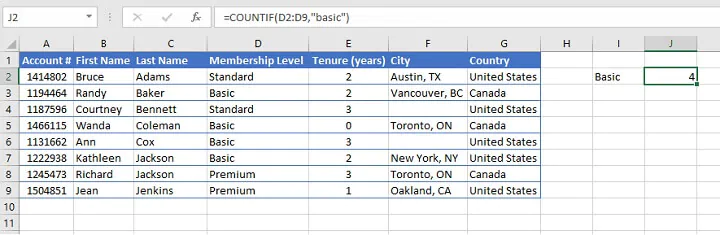As shown above:

• Text criteria must be entered within double quotation marks.
• Text criteria are not case sensitive; therefore, using the criteria of “Basic”, “basic” or “BASIC” would yield the same results.

### Cell reference criteria

We can also point to a cell that has the criteria value by using a cell reference.

In the example below, the criteria refer to the value in cell I2 (Basic). No double quotation marks are used because “I2” is not the literal value being searched for within the range.

````=COUNTIF(D2:D9,I2)`
```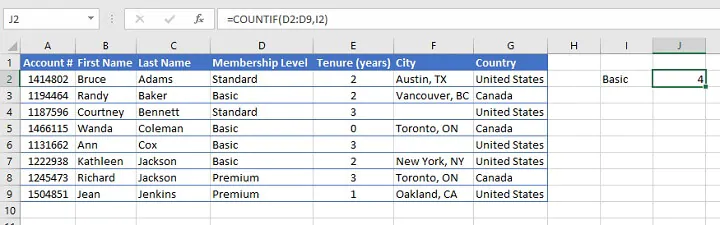### Numeric criteria

Likewise, when using numeric criteria within a COUNTIF formula, no double quotation marks are required. The following formula...

``=COUNTIF(E2:E9,2)``

...will return the number of occurrences of the number “2” in the range E2 to E9.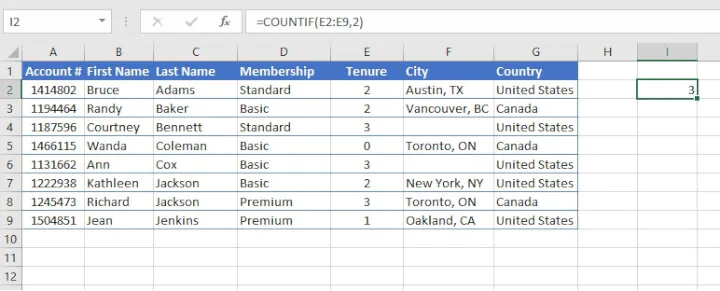### Logical expressions as criteria

The COUNTIF formula makes use of the standard logical mathematical operators (=, >, <). However, the criteria argument defaults to “is equal to” — therefore there is no need to enter the equal sign (=) when stating the “is equal to” criterion.

The following combinations of these operators are also used for even more specific comparisons.

Operator

Meaning

>=

Greater than or equal to

<=

Less than or equal to

<>

Not equal to

#### Logical expression with numeric value

Below is the format to apply the COUNTIF function to determine the number of customers with more than one year of membership.

``=COUNTIF(E2:E9,">1")``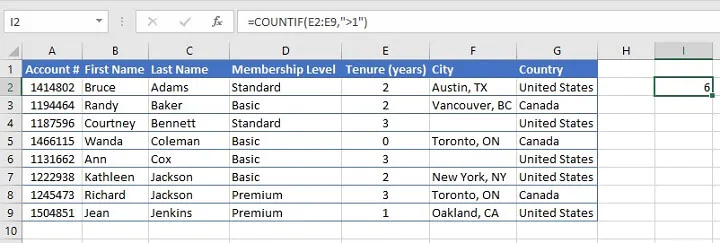Note that both the logical operator > (greater than) and the numeric value are enclosed in double quotes.

Using the format...

``=COUNTIF(E2:E9, “>”&1)``

...would have yielded the same result.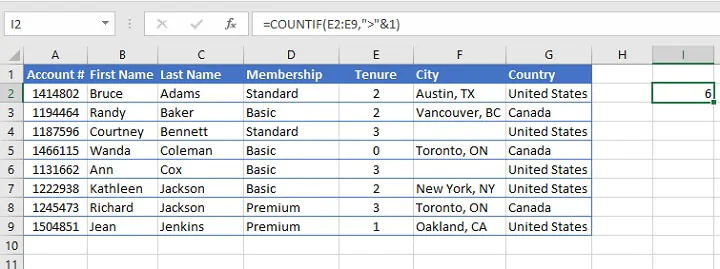#### Logical expression with cell reference

When using logical operators with cell reference criteria, the logical operator itself is enclosed within double quotation marks, followed by an ampersand (&) to concatenate or join the operator to the relevant cell reference.

The following example counts the number of cells greater than the value in cell J1.

``=COUNTIF(\$E2:\$E9,">"&J1)``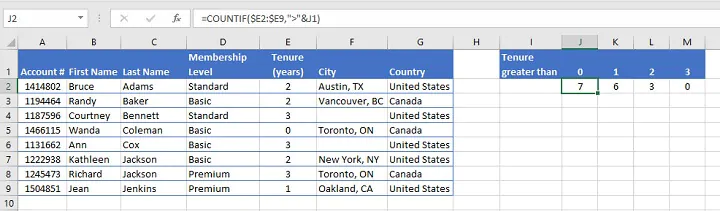There are no quotation marks around the cell reference since “E2” is not the literal value being extracted from the cell range.

In the above example, mixed cell references are used (\$E2:\$E9) to make it easier when copying the formula across from cell J2 to M2. Read more about mixed cell references here.

#### Count blank cells

Applying the above principles, we can also determine the number of blank cells within a range with the format...

``=COUNTIF(range, “”)``

...where the criteria consist of a pair of empty double quotes.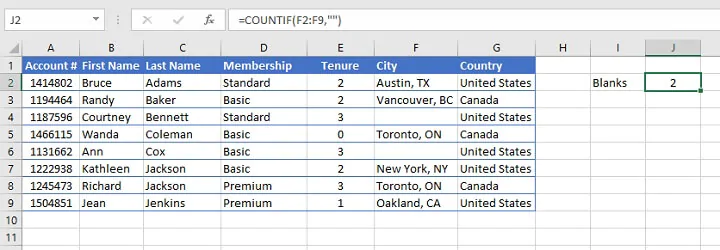Note that the COUNTBLANK function in Excel gives the same result and is just as easy to use.

## Count Booleans

The COUNTIF function can also count Boolean results (TRUE or FALSE). Since Excel translates TRUE into the mathematical value 1, and FALSE into the mathematical value 0, Booleans are treated as numbers rather than text. Therefore, there is no need to use double quotes when our criteria is a Boolean.

This is seen in the following example.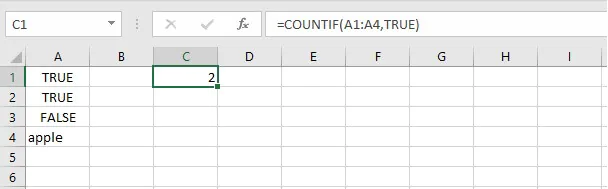## Using COUNTIF with wildcards

A common problem when looking to summarize data is that the data we would like to group is often similar but not identical.

Suppose we want to count the number of Toyota-made cars appearing in the following list. We would not be able to simply use the word “Toyota” as a text criterion since each cell contains unique model names.

Wildcards provide an excellent solution for this problem, and the COUNTIF function supports the use of wildcards to accommodate partial matches.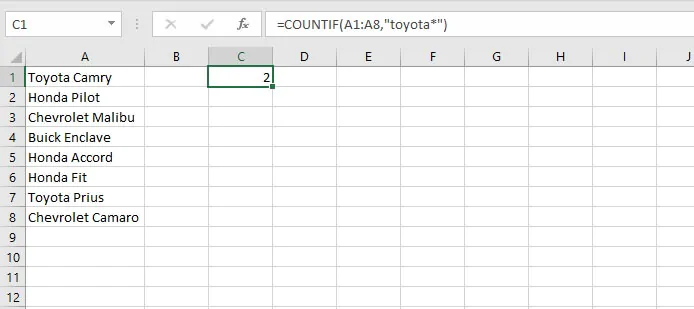Excel counts both occurrences of the word Toyota because the asterisk acts as a placeholder for any characters which may appear after that word.

The COUNTIF function supports the use of wildcards to accommodate partial matches.

Note that wildcards do not work with numeric values.

Wildcard

Meaning

*

Any number or string of unknown characters, or no character

?

A single unknown character

~

Precedes an asterisk or question mark to be used as a literal character

## Multiple criteria

### COUNTIF with OR logic

There may be times when we’d like to count cells within a range that satisfies any one of multiple criteria, like “Toyota” or “Honda”. This scenario is fairly easy to resolve.

We can build a COUNTIF formula that searches for “Toyota*”, and one which searches for “Honda*”, and simply ask Excel to add their results by placing a plus sign (+) between them, as shown below.

``=COUNTIF(A1:A8, “toyota*”)+COUNTIF(A1:A8, “honda*”)``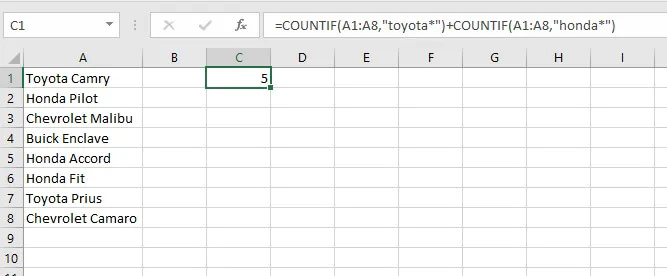The first formula identifies and counts cells in the range A1 to A8 that contain the word “Toyota”, and which may or may not be followed by additional characters.

The second formula identifies and counts cells in the range A1 to A8 that contain the word “Honda”, and which may or may not be followed by additional characters.

The results of both formulas are added and a total of 5 is displayed in cell C1.

### COUNTIF with AND logic

What about cells that must satisfy more than one criteria, like greater than 5 and less than 10?

Using COUNTIF with an AND logic is somewhat less straightforward because we are seeking to isolate cells within a range that meet two or more conditions.

The problem is that the COUNTIF function only works with one criterion at a time, and the AND function only evaluates one cell at a time (it is unable to evaluate entire ranges). Therefore they are incompatible.

The COUNTIFS function exists to accommodate this scenario.

## Useful COUNTIF formats to remember

Here is a handy summary of the formats for the different types of criteria used with the COUNTIF function.

Criteria

Format example

Text value

=COUNTIF(range, “apples”)

Text value with unknown characters before and/or after

=COUNTIF(range, “*apple*”)

Text value followed by a single unknown character

=COUNTIF(range, “apple?”)

Criteria which includes a literal question mark

=COUNTIF(range, “what~?”)

Numeric value

=COUNTIF(range,500)

Greater than or equal to numeric value

=COUNTIF(range, “>=500”)

Equal to cell reference

=COUNTIF(range,D7)

Not equal to value in cell reference

=COUNTIF(range, “<>”&D7)

Satisfies either one of multiple criteria

=COUNTIF(range,criteria) + COUNTIF(range,criteria)

Satisfies multiple criteria

use COUNTIFS function

## Summary

We use the COUNTIF Excel function to identify and count the number of times a value appears in an Excel range. It gives a single output and is therefore useful for summarizing data.

Learn more functions and time-saving techniques with the Microsoft Excel - Basic and Advanced course today. Or start with our free Excel in an Hour course for some formula basics.

Start learning formulas, functions, and time-saving hacks today with this free course!#### Join the Excel conversation on Slack

Ask a question or join the conversation for all things Excel on our Slack channel.Claudia Buckley

Claudia is a project manager and business skills instructor at GoSkills. In her spare time, she reads mystery novels and does genealogy research.